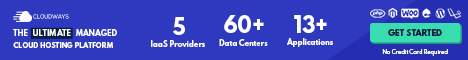# Real GDP versus Nominal GDP

Perhaps you might want to know up front the meaning of these two economic concepts. Well, here's what they mean:

• Real GDP is a measure of the volume of final goods and services, holding prices constant.
• Nominal GDP is the market value of final goods and services evaluated at current year prices.

Because GDP Opens in new window is measured in value terms we have to be careful about interpreting changes over time. To see why, consider interpreting an increase in the total value of coal production from, say, \$80 billion in 2014 to \$100 billion in 2015.

Can we be sure that, because \$100 billion is 25 percent greater than \$80 billion, the amount of coal produced in 2015 was 25 percent greater than the amount produced in 2014?

We can draw this conclusion only if the average price of coal did not change between 2014 and 2015.

In fact, when GDP increases from one year to the next, the increase is due partly to increases in production of goods and services, and partly due to increases in prices.

Because we are mainly interested in GDP as a measure of production, we need a way of separating the price changes from the quantity changes.

## Calculating Real GDP

The ABS Opens in new window separates price changes from quantity changes by calculating a measure of production called real GDP.

Nominal GDP is calculated by summing the current values of final goods and services. Real GDP is a measure of the volume of final goods and services, holding prices constant.

In this sense, real GDP is a measure of the volume of production, rather than the value of production.

To determine by how much the volume of GDP changes from one year to the next, we need to measure the value of GDP in each year using the same unit prices.

It used to be common for most national statistical organizations, including the ABS, to choose a particular year as the base year for prices.

The prices of goods and services in the base year were then used to calculate the value of goods and services in all other years.

For instance, if the base year was 2000, real GDP for 2015 would be calculated by using prices of goods and services from 2000.

By keeping prices constant, we know that changes in real GDP represent changes in the quantity of goods and services produced in the economy Opens in new window.

One drawback to calculating real GDP using base year prices is that, over time, prices change relative to each other.

For example, the prices of mobile phones and computers have fallen dramatically while the prices of most goods and services have risen.

Because this change is not reflected in the fixed prices from the base year, the estimate of real GDP is somewhat distorted.The further away the current year is from the base year, the worse the problem becomes.

To make the calculation of real GDP more accurate, in 1998 the ABS switched to using chain volume measures to estimate real GDP.

The details of calculating real GDP using chain volume measures are more complicated than we need discuss here, but the basic idea is straightforward.

Starting with the previous year as the base year, the ABS takes an average of prices in the current year and prices in the previous year.

It then uses this average to calculate real GDP in the current year. For the next year, the ABS calculates real GDP by taking an average of prices in that year and the previous year, and so on.

This method is known as the annually reweighted chain volume measure Opens in new window. In this way, prices in each year are chained to prices from the previous year, and the distortion from changes in relative prices is minimized.

Holding prices constant means that the purchasing power of a dollar remains the same from one year to the next.

Ordinarily, the purchasing power of the dollar falls every year as price increases reduce the amount of goods and services that a dollar can buy.

Real GDP holds prices constant, which makes it a better measure than nominal GDP of changes in the production of goods and services from one year to the next.

In fact, growth in the economy Opens in new window is almost always measured as growth in real GDP.

If a headline in The Australian Financial Review states, ‘Economy grew 3.5 percent last year’, the article will report that real GDP increased by 3.5 percent during the previous year.